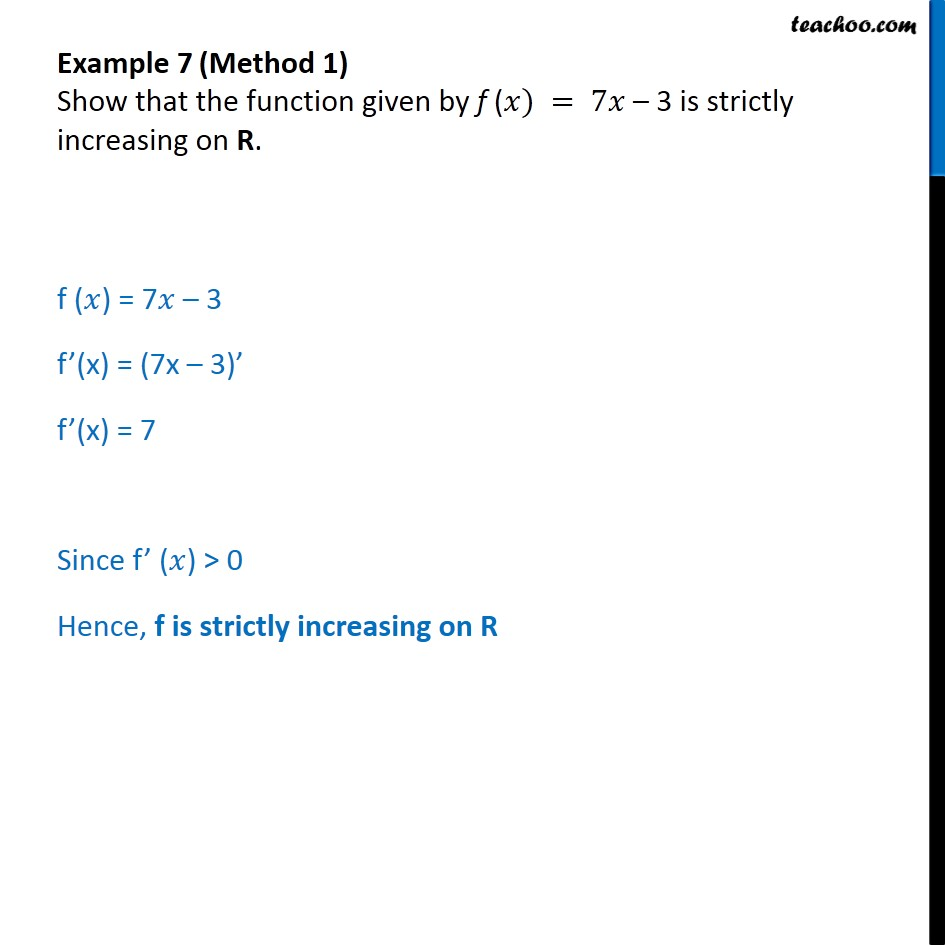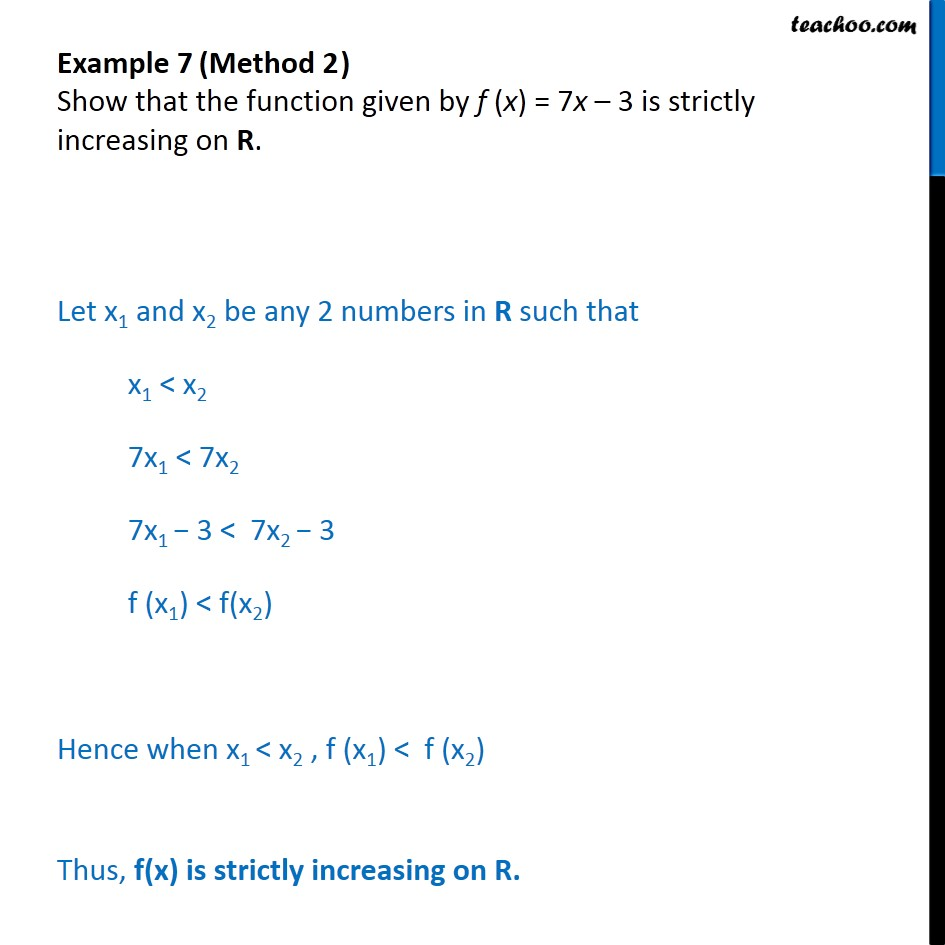1. Chapter 6 Class 12 Application of Derivatives
2. Serial order wise
3. Examples

Transcript

Example 7 (Method 1) Show that the function given by f ( ) = 7 3 is strictly increasing on R. f ( ) = 7 3 f (x) = (7x 3) f (x) = 7 Since f ( ) > 0 Hence, f is strictly increasing on R Example 7 (Method 2 ) Show that the function given by f (x) = 7x 3 is strictly increasing on R. Let x1 and x2 be any 2 numbers in R such that x1 < x2 7x1 < 7x2 7x1 3 < 7x2 3 f (x1) < f(x2) Hence when x1 < x2 , f (x1) < f (x2) Thus, f(x) is strictly increasing on R.

Examples